# NCERT Solutions for Class 9 Maths Exercise 13.9## myCBSEguide App

CBSE, NCERT, JEE Main, NEET-UG, NDA, Exam Papers, Question Bank, NCERT Solutions, Exemplars, Revision Notes, Free Videos, MCQ Tests & more.

NCERT solutions for Class 9 Maths Surface Areas and Volumes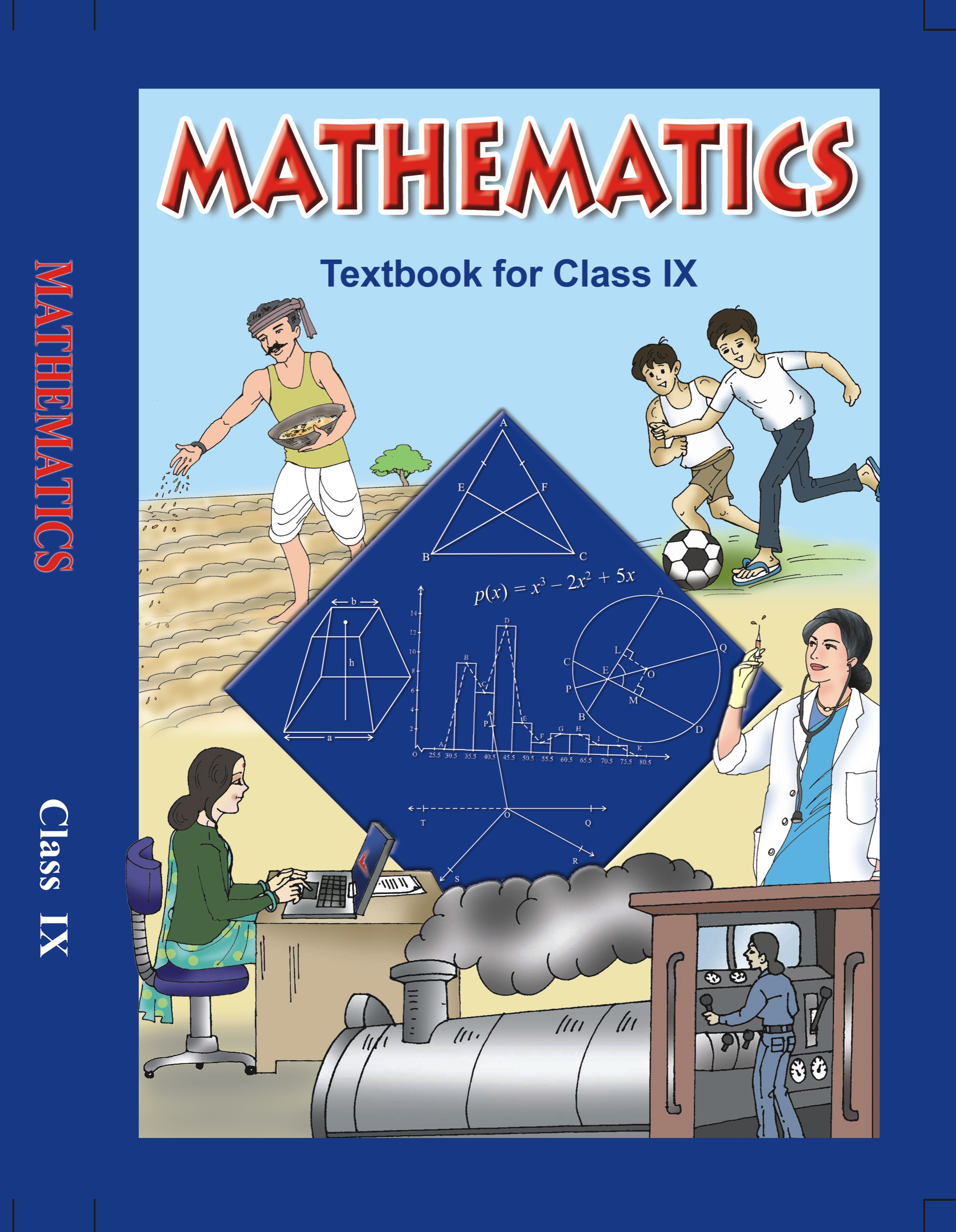## NCERT Solutions for Class 9 Mathematics Surface Areas and Volumes

###### 1.A wooden bookshelf has external dimensions as follows: Height = 110 cm, Depth = 25 cm, Breadth = 85 cm [See fig.]. The thickness of the planks is 5 cm everywhere. The external faces are to be polished and the inner faces are to be painted. If the rate of polishing is 20 paise per cm2 and the rate of painting is 10 paise per cm2, find the total expenses required for polishing and painting the surface of the bookshelf.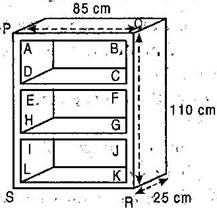Ans. External faces to be polished

= Area of six faces of cuboidal bookshelf – 3 (Area of open portion ABCD)

= 2 (110 x 25 + 25 x 85 + 85 x 110) – 3 (75 x 30)

[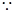AB = 85 – 5 – 5 = 75 cm and AD

=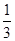x 110 – 5 – 5 – 5 – 5 = 30 cm]

= 2 (2750 + 2125 + 9350) – 3 x 2250

= 2 x 14225 – 6750

= 28450 – 6750

= 21700 cm2

Now, cost of painting outer faces of wodden bookshelf at the rate of 20 paise.

= Rs. 0.20 per cm2 = Rs. 0.20 x 21700 = Rs. 4340

Here, three equal five sides inner faces.

Therefore total surface area

= 3 [ 2 (30 + 75) 20 + 30 x 75] [Depth = 25 – 5 = 20 cm]

= 3 [ 2 x 105 x 20 + 2250] = 3

[ 4200 + 2250]

= 3 x 6450 = 19350 cm2

Now, cost of painting inner faces at the rate of 10 paise i.e. Rs. 0.10 per cm2.

= Rs. 0.10 x 19350 = Rs. 1935

Total expenses required = Rs. 4340 + Rs. 1935 = Rs. 6275

NCERT Solutions for Class 9 Maths Exercise 13.9

###### 2.The front compound wall of a house is decorated by wooden spheres of diameter 21 cm, placed on small supports as shown in figure. Eight such spheres are used for this purpose and are to be painted silver. Each support is a cylinder of radius 1.5 cm and height 7 cm and is to be painted black. Find he cost of paint required if silver paint costs 25 paise per cm2 and black paint costs 5 paise per cm2.Ans. Diameter of a wooden sphere

= 21 cm.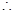Radius of wooden sphere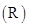=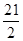cm

And Radius of the cylinder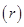= 1.5 cm

Surface area of silver painted part

= Surface area of sphere – Upper part of cylinder for support

=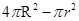=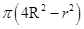=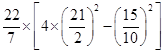=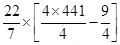=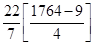=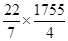= 1378.928 cm2

Surface area of such type of 8 spherical part = 8 x 1378.928

= 11031.424 cm2Cost of silver paint over 1 cm2

= Rs. 0.25Cost of silver paint over 11031.928 cm2 = 0.25 x 11031.928

= Rs. 2757.85

Now, curved surface area of a cylindrical support =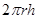=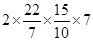= 66 cm2

Curved surface area of 8 such cylindrical supports = 66 x 8 = 528 cm2Cost of black paint over 1 cm2 of cylindrical support = Rs. 0.50Cost of black paint over 528 cm2 of cylindrical support = 0.50 x 528

= Rs. 26.40Total cost of paint required

= Rs. 2757.85 + Rs. 26.4

= Rs. 2784.25

NCERT Solutions for Class 9 Maths Exercise 13.9

###### 3. If diameter of a sphere is decreased by 25% then what percent does its curved surface area decrease?

Ans. Diameter of original sphere

= D = 2RR =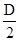Curved surface area of original sphere =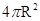==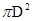According to the question,

Decreased diameter = 25% of D

=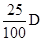=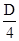Diameter of new sphere =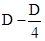=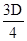Radius of new sphere =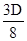Now, curved surface area of new sphere =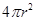=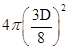=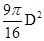Change in curved surface area

=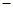=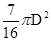Percent change in the curved surface area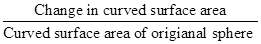x 100

=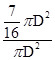x 100 =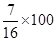= 43.75%

NCERT Solutions for Class 9 Maths Exercise 13.9

###### 4. Sameera wants to celebrate the fifth birthday of her daughter with a party. She bought thick paper to make the conical party caps. Each cap is to have a base diameter of 10 cm and height 12 cm. A sheet of the paper is 25 cm by 40 cm and approximately 82% of the sheet can be effectively used for making the caps after cutting. What is the minimum number of sheets of paper that Sameera would need to buy, if there are to be 15 children at the party?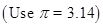Ans. Diameter of base of conical cap

= 10 cm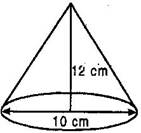Radius of conical cap= 5 cm

Slant height of cone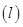=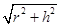=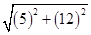=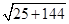=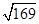= 13 cm

Curved surface area of a cap

=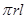= 3.14 x 5 x 13 = 204.1 cm2

Curved surface area of 15 caps

= 15 x 204.1 = 3061.5 cm2

Area of a sheet of paper used for making caps = 25 x 40 = 1000 cm2

82% of sheet is used after cutting

= 82% of 1000 cm2

=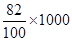= 820 cm2

Number of sheet =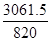= 3.73

Hence 4 sheets area needed .

## NCERT Solutions for Class 9 Maths Exercise 13.9

NCERT Solutions for Class 9 Maths PDF (Download) Free from myCBSEguide app and myCBSEguide website. Ncert solution class 9 Maths includes text book solutions from Mathematics Book. NCERT Solutions for CBSE Class 9 Maths have total 15 chapters. 9 Maths NCERT Solutions in PDF for free Download on our website. Ncert Maths class 9 solutions PDF and Maths ncert class 9 PDF solutions with latest modifications and as per the latest CBSE syllabus are only available in myCBSEguide.

## CBSE app for Class 9

To download NCERT Solutions for Class 9 Maths, Computer Science, Home Science,Hindi ,English, Social Science do check myCBSEguide app or website. myCBSEguide provides sample papers with solution, test papers for chapter-wise practice, NCERT solutions, NCERT Exemplar solutions, quick revision notes for ready reference, CBSE guess papers and CBSE important question papers. Sample Paper all are made available through the best app for CBSE students and myCBSEguide website.Test Generator

Create question papers online with solution using our databank of 5,00,000+ questions and download as PDF with your own name & logo in minutes.

### 4 thoughts on “NCERT Solutions for Class 9 Maths Exercise 13.9”

1. Gud…..

2. tnx you are a good person

3. tnx

4. Thanks for kind support. I like this site as well as its app.